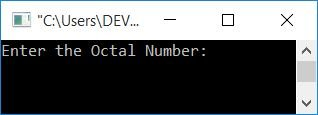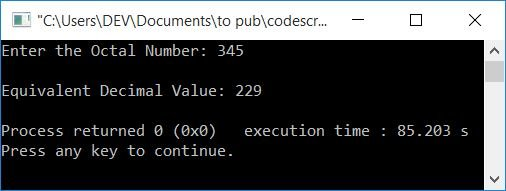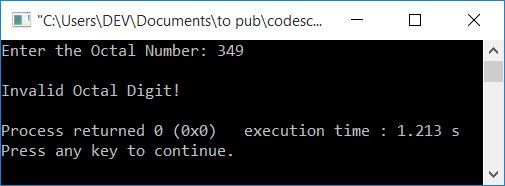# C++ Program to Convert Octal to Decimal

In this article, you will learn and get code for octal to decimal conversion in C++. The octal to decimal conversion program is designed both with and without functions.

But before starting the program, if you're not aware of the simple formula and steps used for the conversion, you can refer to the octal to decimal formula to get all the required information.

## Octal to Decimal in C++

To convert an octal number to a decimal number in C++ programming, you have to ask the user to enter the octal number and then convert it into its equivalent decimal value. Print the equivalent decimal value on the output as shown in the program given below.

The question is: write a program in C++ that converts an octal number to a decimal number. Here is its answer:

```#include<iostream>
#include<math.h>
using namespace std;
int main()
{
int octalNum, decimalNum=0, i=0, rem;
cout<<"Enter the Octal Number: ";
cin>>octalNum;
while(octalNum!=0)
{
rem = octalNum%10;
decimalNum = decimalNum + (rem*pow(8,i));
i++;
octalNum = octalNum/10;
}
cout<<"\nEquivalent Decimal Value: "<<decimalNum;
cout<<endl;
return 0;
}```

This program was built and runs under the Code::Blocks IDE. Here is its sample run:Now supply the input as any octal number, say 345, and press the ENTER key to convert and print its equivalent decimal value as shown here in the snapshot of sample output given below:The dry run of the above program with octal input 345 goes like this:

• Initial values are as follows: decimalNum=0, i=0.
• And when the user enters an octal number as input, say 345, then it gets stored in the octalNum variable. So octalNum=345
• Now the condition octalNum!=0 or 345!=0 evaluates to be true, therefore program flow goes inside the while loop.
• There, octalNum%10, 345%10, or 5 is set to rem. So rem=5
• And decimalNum + (rem*pow(8,i)) or 0+(5*8i) or 0+(5*80) or 5*1 or 5 gets initialized to decimalNum. So decimalNum=5
• The value of i gets incremented. So i=1
• And octalNum/10, 345/10, or 34 is set to octalNum. So octalNum=34
• Program flow goes back and evaluates the condition of the while loop again.
• The execution of the while continues until its condition evaluates to be false.
• Before its condition evaluates to be false, we'll get values (after each evaluation) as follows:
1. rem=5, decimalNum=5, i=1, octalNum=34
2. rem=4, decimalNum=37, i=2, octalNum=3
3. rem=3, decimalNum=229, i=3, octalNum=0
• Now print the value of decimalNum (229), which will be the decimal equivalent of the given octal number (345).

### Octal to decimal conversion in C++ without using the pow() function

To create the same program as the previous one without using the pow() function, just replace the following statement:

```decimalNum = decimalNum + (rem*pow(8,i));
i++;```

with the statement given below:

```decimalNum = decimalNum + (rem*mul);
mul = mul*8;```

Note: Don't forget to declare the variable mul at the start of the program. and initialize its initial value as 1.

Remove the declaration and initialization of variable i from the program. Also remove the header file, math.h. Everything else will remain the same.

### Program to deal with invalid octal input

The octal number has an 8 as its base. Therefore, it has a total of eight digits, which are from 0 to 7. Other digits, like 8 and 9, are not valid octal digits. Therefore, if the user enters a number that contains 8 or 9, Then here is the program to deal with:

```#include<iostream>
using namespace std;
int main()
{
int octalNum, rem, temp, chk=0;
cout<<"Enter the Octal Number: ";
cin>>octalNum;
temp = octalNum;
while(temp!=0)
{
rem = temp%10;
if(rem>=8)
{
chk++;
break;
}
temp = temp/10;
}
if(chk==0)
{
int decimalNum=0, mul=1;
while(octalNum!=0)
{
rem = octalNum%10;
decimalNum = decimalNum + (rem*mul);
mul = mul*8;
octalNum = octalNum/10;
}
cout<<"\nEquivalent Decimal Value: "<<decimalNum;
}
else
cout<<"\nInvalid Octal Digit!";
cout<<endl;
return 0;
}```

Here is a sample run with 349 as an octal number as user input. Because it contains 9 (the third digit of 349) and 9 is an invalid octal digit, here is the output you will see:### Using a Function to Convert Octal to Decimal in C++

This is the last program on octal to decimal conversion in C++. It is created using a user-defined function, octalToDecimal(). The function takes an octal number as its argument and returns either its equivalent decimal value or 0.

It returns 0 if there is an invalid octal digit in the octal number that was entered. Otherwise, it returns the decimal value that the octal number would be in decimal.

```#include<iostream>
using namespace std;
int OctalToDecimal(int);
int main()
{
int octalNum, decimalNum;
cout<<"Enter the Octal Number: ";
cin>>octalNum;
decimalNum = OctalToDecimal(octalNum);
if(decimalNum==0)
cout<<"\nInvalid Octal Digit!";
else
cout<<"\nEquivalent Decimal Value: "<<decimalNum;
cout<<endl;
return 0;
}
int OctalToDecimal(int octalNum)
{
int temp, rem, chk=0;
temp = octalNum;
while(temp!=0)
{
rem = temp%10;
if(rem>=8)
{
chk++;
break;
}
temp = temp/10;
}
if(chk==0)
{
int decimalNum=0, mul=1;
while(octalNum!=0)
{
rem = octalNum%10;
decimalNum = decimalNum + (rem*mul);
mul = mul*8;
octalNum = octalNum/10;
}
return decimalNum;
}
else
return 0;
}```

This program produces the same output as the previous program.

#### The same program in different languages

C++ Quiz

« Previous Program Next Program »